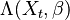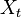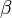# Default Intensity

## Definition

A Default Intensity is any function of the form$\Lambda(X_t , \beta)$, where:

•$X_t$ is a list of
• firm-specific and macroeconomic default covariates, some of which might suggested by structural theories,
• unobservable covariates, and
•$\Lambda(X_t , \beta)$ is an ad-hoc function, not necessarily based on a theory of the firm, depending on a parameter vector$\beta$ to be estimated.

In the context of Credit Risk modelling the default intensity denotes the rate of default at any time assuming the obligor has survived up to that point. It is closely related to the concept of a Hazard Rate, with the intensity name being used more widely in the context of probability / stochastic models literature and the hazard rate being used more widely in the context of statistical literature and survival analysis.